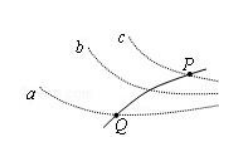$\text{A.}$ 带电粒子通过 P 点时的动能比通过 Q 点时小 $\text{B.}$ 带电粒子一定是从 P 点向 Q 点运动 $\text{C.}$ 带电粒子通过 P 点时的加速度比通过 Q 点时 $\text{D.}$ 三个等势面中，等势面 a 的电势最

A